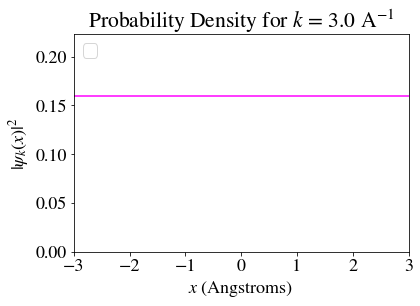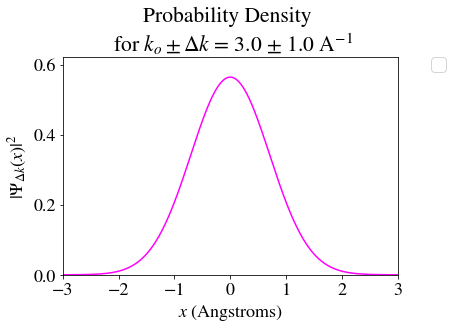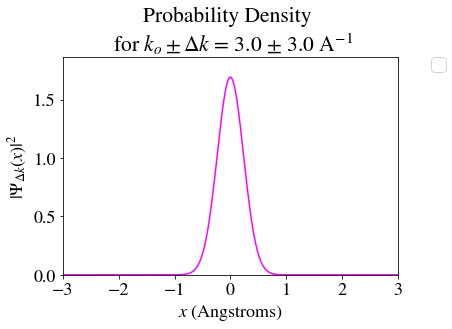# 2.1: Describing Matter-Waves

•• Valeria D. Kleiman
• University of Florida
$$\newcommand{\vecs}{\overset { \rightharpoonup} {\mathbf{#1}} }$$ $$\newcommand{\vecd}{\overset{-\!-\!\rightharpoonup}{\vphantom{a}\smash {#1}}}$$$$\newcommand{\id}{\mathrm{id}}$$ $$\newcommand{\Span}{\mathrm{span}}$$ $$\newcommand{\kernel}{\mathrm{null}\,}$$ $$\newcommand{\range}{\mathrm{range}\,}$$ $$\newcommand{\RealPart}{\mathrm{Re}}$$ $$\newcommand{\ImaginaryPart}{\mathrm{Im}}$$ $$\newcommand{\Argument}{\mathrm{Arg}}$$ $$\newcommand{\norm}{\| #1 \|}$$ $$\newcommand{\inner}{\langle #1, #2 \rangle}$$ $$\newcommand{\Span}{\mathrm{span}}$$ $$\newcommand{\id}{\mathrm{id}}$$ $$\newcommand{\Span}{\mathrm{span}}$$ $$\newcommand{\kernel}{\mathrm{null}\,}$$ $$\newcommand{\range}{\mathrm{range}\,}$$ $$\newcommand{\RealPart}{\mathrm{Re}}$$ $$\newcommand{\ImaginaryPart}{\mathrm{Im}}$$ $$\newcommand{\Argument}{\mathrm{Arg}}$$ $$\newcommand{\norm}{\| #1 \|}$$ $$\newcommand{\inner}{\langle #1, #2 \rangle}$$ $$\newcommand{\Span}{\mathrm{span}}$$$$\newcommand{\AA}{\unicode[.8,0]{x212B}}$$

## Wavefunctions

In 1926 Max Born proposed a probabilistic description of the wave behavior of a particle. To understand the Born Interpretation, we can use an analogy with classical waves: the wavefunction $$\Psi(x,t)$$ is a function that describes the wave properties of a particle, and the behavior of this wave in space and time is governed by a wave equation.

Born described the wavefunction $$( \Psi(x,y,z,t) )$$ as a Probability Amplitude, and the square of the absolute value of this amplitude, $$|\Psi(x,y,z,t)|^{2}$$ , as a Probability Density. Whereas the value of the Amplitude has no physical meaning, the Probability Density is proportional to the probability of finding a particle/wave in a point in space at the time t. When doing an actual measurement, we always measure a specific volume of space, thus the Probability to find a particle in a given volume will be given by $$|\Psi(x,y,z,t)|^2 \Delta V$$ or, to be more precise

$P= \int | \Psi(x,y,z,t)|^{2} d\tau$

where $$d\tau$$ is the volume element in three-dimensional space

From this interpretation, there are a few things that we can learn

If the value of $$| \Psi(x_1,y_1,z_1,t)|^{2}$$ is larger than $$| \Psi(x_2,y_2,z_2,t)|^{2}$$ then the probability to find the particle at position $$x_1,y_1,z_1$$ is larger than a position $$x_2,y_2,z_2$$.

If the value of $$| \Psi(x_1,y_1,z_1,t)|^{2}$$ is equal to the value of $$| \Psi(x_2,y_2,z_2,t)|^{2}$$ then the probability to find the particle at position $$(x_1,y_1,z_1)$$ is equal than a position $$(x_2,y_2,z_2)$$ at time $$t$$.

As a consequence of the probabilistic interpretation there are some important properties for the wavefunction

For each point in space $$x_1,y_1,z_1)$$, there is a single value of $$| \Psi(x_1,y_1,z_1,t)|^{2}$$, since it is not possible to have two different probabilities to find the particle in one point in space at a given time.

If we know that the particle exists within a limited space, then the total probability to find it within that space must be unity. $\int^{\infty}_{-\infty} | \Psi(x_1,t)|^{2} =1$

There are points in space where the wavefunction changes sign and thus $$|\Psi(x_1,y_1,z_1,t)|^{2} =0$$. These points are called Nodes. The probability to find the particle at a node is null.

## The simplest particle-wave: a Free Electron

We start by describing a free electron: a particle that is not under the influence of a potential.
As any other particle, the state of a ***Free electron*** is described with a wavefunction $$\psi(x)$$ and we want to answer the question: "Where is the electron?"

Like any other free particle moving, this electron will have momentum $$p = \frac{h}{\lambda}$$. If the electron is described by a single wave, then it has a single value of the momentum $$p = h k$$, where $$k=\frac{1}{\lambda}$$ is called the wave-vector.

We can evaluate the probability of finding the electron at a given point in space, or, even better, we can plot the probability density as a function of position for a range of x values, to see what is the probability to find in different places at a given time.

Example $$\PageIndex{1}$$ : A free electron with fully defined momentum

For an electron with a momentum $$p= 3.16 \cdot 10^{-24} \frac{kg\cdot m}{s}$$ the wave-vector is $$k=3.0 \ Å^{-1}$$ the probability density $$| \psi(x)|^{2}$$ as a function of position is shown belowThis plot shows that the probability to find a free electron is exactly the same for ALL values of $$x$$, i.e. $$| \psi(-3)|^{2} = | \psi(0)|^{2} =| \psi(-1.2)|^{2}$$, etc ) . Even though we know exactly the momentum of the electron, we know nothing specific about its position. At the time of the measurement, it has the same probability of being found at any possible point in this one-dimensional space.

Example $$\PageIndex{2}$$: A free electron with some uncertainty in the momentum

What happens if instead of having the free electron with an exact value of momentum, we allow the momentum to have a range of values (uncertainty in the momentum $$= \Delta p$$)?

For an electron with wave-vector momentum $$k=3.0 \pm \ 1.0 Å^{-1}$$ (or momentum $$p = 3.16 \pm 1.05 \cdot 10^{-24} \frac{kg\cdot m}{s}$$, we can evaluate the values of the probability density $$| \psi(x)|^{2}$$ as a function of position as shown belowNow, the probability to find the electron at $$x=0\ Å$$ is larger than at $$x=1.2\ Å$$ and much larger than at $$x=3 \ Å$$. This is telling us that it is very likely to find the electron near $$x=0.$$, but it will be very unlikely to find the electron at large values of $$x$$. We can also say that the probability to find the particle somewhere between $$x=-2 \ Å$$ and $$x=2 \ Å$$ is almost 100%, whereas the probability to find it at values of $$x \leq -2 \ Å$$ or $$x \geq 3 \ Å$$ is almost 0.

Example $$\PageIndex{3}$$: A free electron with a large uncertainty in the momentum

What happens if we now allow the electron to have a very large range of values of momenta (very large $$\Delta p$$ )?

For an electron with wave-vector momentum $$k=3.0 \pm \ 3.0 Å^{-1}$$ (or moment with any value from $$p =0$$ to $$7.32 \cdot 10^{-24} \frac{kg\cdot m}{s}$$, we can evaluate the values of the probability density $$| \psi(x)|^{2}$$ as a function of position as shown belowNow the probability to find the electron at $$x=0\ Å$$ is much larger than at $$x=1.2 or x =3 \ Å$$. When the particle can have these varying values of momenta, we have almost (not quite, but close to) 100% probability to find it somewhere between $$x=-1 \ Å$$ and $$x=1 \ Å$$, meaning that the position of the particle is much more certain than before.
Even though we now know less about the exact value of the momentum, we know a bit more precisely where to look for the electron.

Obervation:

By plotting the probability density for the free electron we observe that as the uncertainty on the momentum increases (larger $$\Delta p$$, the uncertainty in the position of the electron decreases. This relationship is described by Heisenberg Uncertainty Principle.

This page titled 2.1: Describing Matter-Waves is shared under a not declared license and was authored, remixed, and/or curated by Valeria D. Kleiman.